### Comparing Quantities Using Proportion Class 8th Mathematics AP Board Solution

##### Question 1.Sudhakar borrows Rs. 15000 from a bank to renovate his house. He borrows the money at 9% p.a. simple interest over 8 years. What are his monthly repayments?Answer:Principal (P) = Rs. 15000Time period (T) = 8Rate of interest (R) = 9%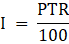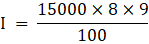I = Rs. 10800∴ Interest for 8 years is Rs. 10800Amount to be paid at the end of 8 years = Principal + interestAmount = 15000+10800= 25800∴ Amount to be paid at the end of 8 years is Rs. 25800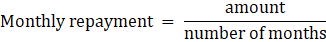⇒ monthly repayment =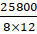= 268.75∴ Sudhakar pays Rs. 268.75 monthly.Question 2.A TV was bought at a price of Rs. 21000. After 1 year the value of the TV was depreciated by 5% (Depreciation means reduction of the value due to use and age of the item). Find the value of the TV after 1 year.Answer:Cost price of TV = Rs. 21000Depreciation = 5%Depreciation after 1 year = 5% of 21000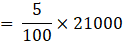∴ Depreciation after 1 year = Rs. 1050Value of TV after 1 year = cost price – depreciation= 21000 – 1050= Rs. 19950∴The value of TV after 1 year is Rs. 19,950Question 3.Find the amount and the compound interest on Rs. 8000 at 5% per annum, for 2 years compounded annually.Answer:Principal (P) = Rs. 8000Time period (n) = 2Rate of interest (R) = 5%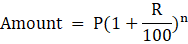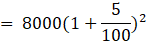= 8000×1.052= 8820∴ Amount = Rs. 8820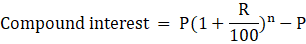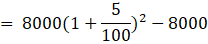= (8000×1.052)-8000= 8820-8000= 820∴ Compound Interest = Rs. 820Question 4.Find the amount and the compound interest on Rs. 6500 for 2 years, compounded annually, the rate of interest being 5% per annum during the first year and 6% per annum during the second year.Answer:Principal (P) for 1st year = Rs. 6500Rate of interest (R) for first year = 5%Interest on first year = 5% of 6500=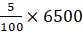= 325∴ Interest for 1st year = Rs. 325Principal (P) for second year = Principal (P) for 1st year + Interest for 1st yearPrincipal (P) for second year = Rs. 6500 + Rs. 325 = Rs. 6825Rate of interest (R) for second year = 6%Interest on second year = 6% of 6825=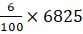= 409.5∴ Interest for 2nd year = Rs. 409.5Total interest = Rs. 325+Rs. 409.5 = Rs. 734.5Amount at second year = Principal (P) for 2nd year + Interest for 2nd year= 6825+409.5= 7234.5∴ Amount at second year = Rs. 7234.5Question 5.Prathibha borrows Rs. 47000 from a finance company to buy her first car. The rate of simple interest is 17% and she borrows the money over a 5 year period.Find:(a) How much amount Prathibha should repay the finance company at the end of five years.(b) her equal monthly repayments.Answer:(a) Principal (P) = Rs. 47000Time period (T) = 5Rate of interest (R) = 17%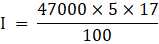I = Rs. 39950∴ Interest for 5 years is Rs. 39950Amount at the end of 5 years = Principal + interestAmount = 47000+39950= 86950∴ Amount at the end of 5 years is Rs. 86950(b)⇒ monthly repayment =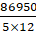= 1449.17∴ prathibha’s monthly repayment isRs. 1449.17.Question 6.The population of Hyderabad was 68,09,000 in the year 2011. If it increases at the rate of 4.7% per annum. What will be the population at the end of the year 2015.Answer:The population of Hyderabad (P) = 68,09,000Time period (n) = 2015-2011 = 4Rate of interest (R) = 4.7%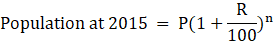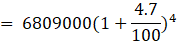= 6809000×1.0474= 81821994∴ The population of Hyderabad at the end of 2015 = 8,18,21,994Question 7.Find Compound interest paid when a sum of Rs. 10000 is invested for 1 year and 3 months at 8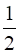% per annum compounded annually.Answer:Principal (P) = Rs. 10000Rate of interest (R) = 8.5%Time period (T) = 1year 3 monthsFor T = 1 year,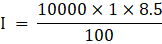I = Rs. 850∴ Interest for 1 year is Rs. 850Amount at the end of 1 year = Principal + interestAmount = 10000+850= 10850∴ Amount at the end of 1 year is Rs. 10850For T = 3 months =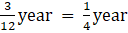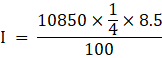I = Rs. 230.56∴ Interest for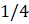year is Rs. 230.56Amount at the end ofyear = Principal + interestAmount = 10850+230.56= 11080.56∴ Amount at the end ofyear is Rs. 11080.56Total interest for 1.3 year = Interest for 1 year + Interest foryear= 850+230.56= 1080.56∴ The compound interest paid is Rs. 1080.56Question 8.Arif took a loan of Rs. 80,000 from a bank. If the rate of interest is 10% per annum, find the difference in amounts he would be paying after 1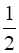years, if the interest is compounded annually and compounded half yearly.Answer:For compounded AnnuallyPrincipal (P) = Rs. 80000Time period (n) = 1Rate of interest (R) = 10%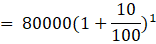= 80000×1.053= 88000∴ Amount for 1 year = Rs. 88000Interest for remaining 6 months =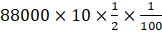= 4400∴ Amount for 1.5 years = Rs. 88000+4400 = Rs. 92400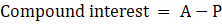= 92400-80000∴ Compound Interest = Rs. 12400For compounded half yearlyPrincipal (P) = Rs. 80000Time period (n) = 3Rate of interest (R) for half year = 10%×= 5%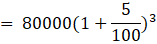= 80000×1.053= 92610∴ Amount = Rs. 92610= 92610-80000∴ Compound Interest = Rs. 12610∴ the difference in amounts = amount for For compounded half yearly - amount for For compounded annually= 92610-92400= Rs. 210∴ The difference in amounts is Rs. 210Question 9.I borrowed Rs. 12000 from Prasad at 6% per annum simple interest for 2 years. Had I borrowed this sum at 6% per annum compounded annually, what extra amount would I have to pay?Answer:Principal (P) = Rs. 12000Rate of interest (R) = 6%Time period (T) = 2years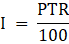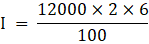I = Rs. 1440∴ Interest for 2 years is Rs. 1440This sum to be borrowed at 6% per annum compounded annually,Principal (P) = Rs. 12000Rate of interest (R) = 6%Time period (n) = 2years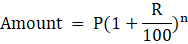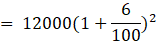= 12000×1.062= 13483.2∴ Amount = Rs. 13483.2= 13483.2-12000∴ Compound Interest = Rs. 1483.2∴ The difference in interest = 1483.2 - 1440= Rs. 43.2∴ The difference in interest is Rs. 43.2Question 10.In a laboratory the count of bacteria in a certain experiment was increasing at the rate of 2.5% per hour. Find the bacteria at the end of 2 hours if the count was initially 5, 06,000Answer:Principal (P) = 506000Rate of interest (R) = 2.5%Time period (n) = 2hours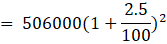= 506000×1.0252= 531616.25∴ The number of the bacteria at the end of 2 hours is 531616(approximately)Question 11.Kamala borrowed Rs. 26400 from a bank to buy a scooter at a rate of 15% per annum compounded yearly. What amount will she pay at the end of 2 years and 4 months to clear the loan?Answer:Principal (P) = 26400Rate of interest (R) = 15%Time period (T) = 2 years and 4 monthsAmount for 2 years,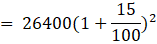= 26400×1.152= Rs. 34914∴ Amount for 2 year = Rs. 34914Interest for remaining 4 months =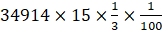= 1745.70∴ Total amount for 2 years and 4 months = Rs. 34914 + 1745.70= Rs. 36659.70∴ The total amount to clear the loan is Rs. 36659.70Question 12.Bharathi borrows an amount of Rs. 12500 at 12% per annum for 3 years at a simple interest and Madhuri borrows the same amount for the same time period at 10% per annum, compounded annually. Who pays more interest and by how much?Answer:Principal (P) = Rs. 12500Rate of interest (R) = 12%Time period (T) = 3yearsInterest paid by bharathi,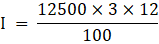I = Rs. 4500∴ Interest paid by bharathi is Rs. 4500Amount paid by madhuri,Principal (P) = Rs. 12500Rate of interest (R) = 10%Time period (n) = 3years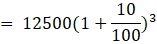= 12500×1.13= Rs. 16637.5∴ Amount paid by madhuri isRs. 16637.5Interest = A-P= Rs. 16637.5 – 12500= Rs. 4137.5∴ Interest paid by madhuri is Rs. 4137.5On comparing the interests paid by bharathi and madhuri,4500-4137.5 = 362.5∴ Bharathi paid Rs.362.5 more than by madhuri.Question 13.Machinery worth Rs. 10000 depreciated by 5%. Find its value after 1 year.Answer:Principal (P) = 10000Depreciation (R) = 5%Time period (n) = 1 year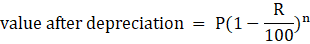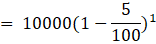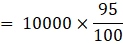= Rs. 9500∴The value of machinery after 1 year is Rs. 9500Question 14.Find the population of a city after 2 years which is at present 12 lakh, if the rate of increase is 4%.Answer:Present population (P) = 12 lakhRate of interest (R) = 4%Time period (n) = 2years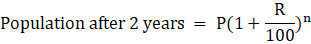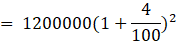= 1200000×1.042= 1297920∴ The population of a city after 2 years is 1297920Question 15.Calculate compound interest on Rs. 1000 over a period of 1 year at 10% per annum, if interest is compounded quarterly?Answer:Principal (P) = 1000Rate of interest (R) = 10%Time period (n) = 1 yearFor quarterly, n = 4Rate of interest (R) for quarterly =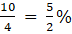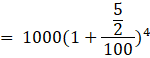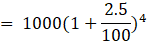= 1000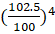= 1103.81∴ Amount = Rs. 1103.81Interest = A-P= 1103.81-1000= 103.81∴ compound interest = Rs. 103.81

PDF FILE TO YOUR EMAIL IMMEDIATELY PURCHASE NOTES & PAPER SOLUTION. @ Rs. 50/- each (GST extra)

HINDI ENTIRE PAPER SOLUTION

MARATHI PAPER SOLUTION

SSC MATHS I PAPER SOLUTION

SSC MATHS II PAPER SOLUTION

SSC SCIENCE I PAPER SOLUTION

SSC SCIENCE II PAPER SOLUTION

SSC ENGLISH PAPER SOLUTION

SSC & HSC ENGLISH WRITING SKILL

HSC ACCOUNTS NOTES

HSC OCM NOTES

HSC ECONOMICS NOTES

HSC SECRETARIAL PRACTICE NOTES

# 2019 Board Paper Solution

HSC ENGLISH SET A 2019 21st February, 2019

HSC ENGLISH SET B 2019 21st February, 2019

HSC ENGLISH SET C 2019 21st February, 2019

HSC ENGLISH SET D 2019 21st February, 2019

SECRETARIAL PRACTICE (S.P) 2019 25th February, 2019

HSC XII PHYSICS 2019 25th February, 2019

CHEMISTRY XII HSC SOLUTION 27th, February, 2019

OCM PAPER SOLUTION 2019 27th, February, 2019

HSC MATHS PAPER SOLUTION COMMERCE, 2nd March, 2019

HSC MATHS PAPER SOLUTION SCIENCE 2nd, March, 2019

SSC ENGLISH STD 10 5TH MARCH, 2019.

HSC XII ACCOUNTS 2019 6th March, 2019

HSC XII BIOLOGY 2019 6TH March, 2019

HSC XII ECONOMICS 9Th March 2019

SSC Maths I March 2019 Solution 10th Standard11th, March, 2019

SSC MATHS II MARCH 2019 SOLUTION 10TH STD.13th March, 2019

SSC SCIENCE I MARCH 2019 SOLUTION 10TH STD. 15th March, 2019.

SSC SCIENCE II MARCH 2019 SOLUTION 10TH STD. 18th March, 2019.

SSC SOCIAL SCIENCE I MARCH 2019 SOLUTION20th March, 2019

SSC SOCIAL SCIENCE II MARCH 2019 SOLUTION, 22nd March, 2019

XII CBSE - BOARD - MARCH - 2019 ENGLISH - QP + SOLUTIONS, 2nd March, 2019

# HSCMaharashtraBoardPapers2020

(Std 12th English Medium)

HSC ECONOMICS MARCH 2020

HSC OCM MARCH 2020

HSC ACCOUNTS MARCH 2020

HSC S.P. MARCH 2020

HSC ENGLISH MARCH 2020

HSC HINDI MARCH 2020

HSC MARATHI MARCH 2020

HSC MATHS MARCH 2020

# SSCMaharashtraBoardPapers2020

(Std 10th English Medium)

English MARCH 2020

HindI MARCH 2020

Hindi (Composite) MARCH 2020

Marathi MARCH 2020

Mathematics (Paper 1) MARCH 2020

Mathematics (Paper 2) MARCH 2020

Sanskrit MARCH 2020

Sanskrit (Composite) MARCH 2020

Science (Paper 1) MARCH 2020

Science (Paper 2)

Geography Model Set 1 2020-2021

MUST REMEMBER THINGS on the day of Exam

Are you prepared? for English Grammar in Board Exam.

Paper Presentation In Board Exam

How to Score Good Marks in SSC Board Exams

Tips To Score More Than 90% Marks In 12th Board Exam

How to write English exams?

How to prepare for board exam when less time is left

How to memorise what you learn for board exam

No. 1 Simple Hack, you can try out, in preparing for Board Exam

How to Study for CBSE Class 10 Board Exams Subject Wise Tips?

JEE Main 2020 Registration Process – Exam Pattern & Important Dates

NEET UG 2020 Registration Process Exam Pattern & Important Dates

How can One Prepare for two Competitive Exams at the same time?

8 Proven Tips to Handle Anxiety before Exams!